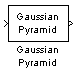# Gaussian Pyramid

Perform Gaussian pyramid decomposition

•Libraries:
Computer Vision Toolbox / Transforms

## Description

The Gaussian Pyramid block computes Gaussian pyramid reduction or expansion to resize an image. The image reduction process involves lowpass filtering and then downsampling the image pixels. The image expansion process involves upsampling and then lowpass filtering the image pixels. You can also use this block to build a Laplacian pyramid.

## Ports

### Input

expand all

Input image, specified as a scalar, a vector, an M-by-N matrix, or an M-by-N-by-C array. C is the number of color channels in the input image. To specify this input as a scalar or vector, you must set the Operation parameter to `Expand`.

Data Types: `single` | `double` | `int8` | `int16` | `int32` | `uint8` | `uint16` | `uint32` | `fixed point`

### Output

expand all

Output image, returned as a scalar, a vector, a matrix, or an array. The dimensions of the output image are determined by the Operationand Pyramid level parameter values. For this port to return a scalar or vector, you must set the Operation parameter to `Reduce`.

Data Types: `single` | `double` | `int8` | `int16` | `int32` | `uint8` | `uint16` | `uint32` | `Boolean` | `fixed point`

## Parameters

expand all

Select the Gaussian pyramid operation as one of these methods:

• `Reduce` — The block applies a lowpass filter and then downsamples the input image.

• `Expand` — The block upsamples and then applies a lowpass filter to the input image.

Specify the factor of 2 by which to upsample or downsample each dimension of the image.

For an M-by-N input image, if you set the Operation parameter to `Reduce` and the Pyramid level to `1`, the dimensions of the output image are `ceil(M/2)`-by-`ceil(N/2)`.

For an M-by-N input image, if you set the Operation parameter to `Expand`, the dimensions of the output image are [(M-1)2l+1]-by-[(N-1)2l+1], where the Pyramid level parameter l must be a positive integer.

Specify the lowpass filter coefficient source as ```Default separable filter [1/4-a/2 1/4 a 1/4 1/4-a/2]``` or ```Specify via dialog```.

Specify the separable filter coefficients as a vector.

Specify the hidden separable filter coefficients as a vector.

## Block Characteristics

 Data Types `double` | `fixed point` | `integer` | `single` Multidimensional Signals `yes` Variable-Size Signals `yes`

## Version History

Introduced before R2006a